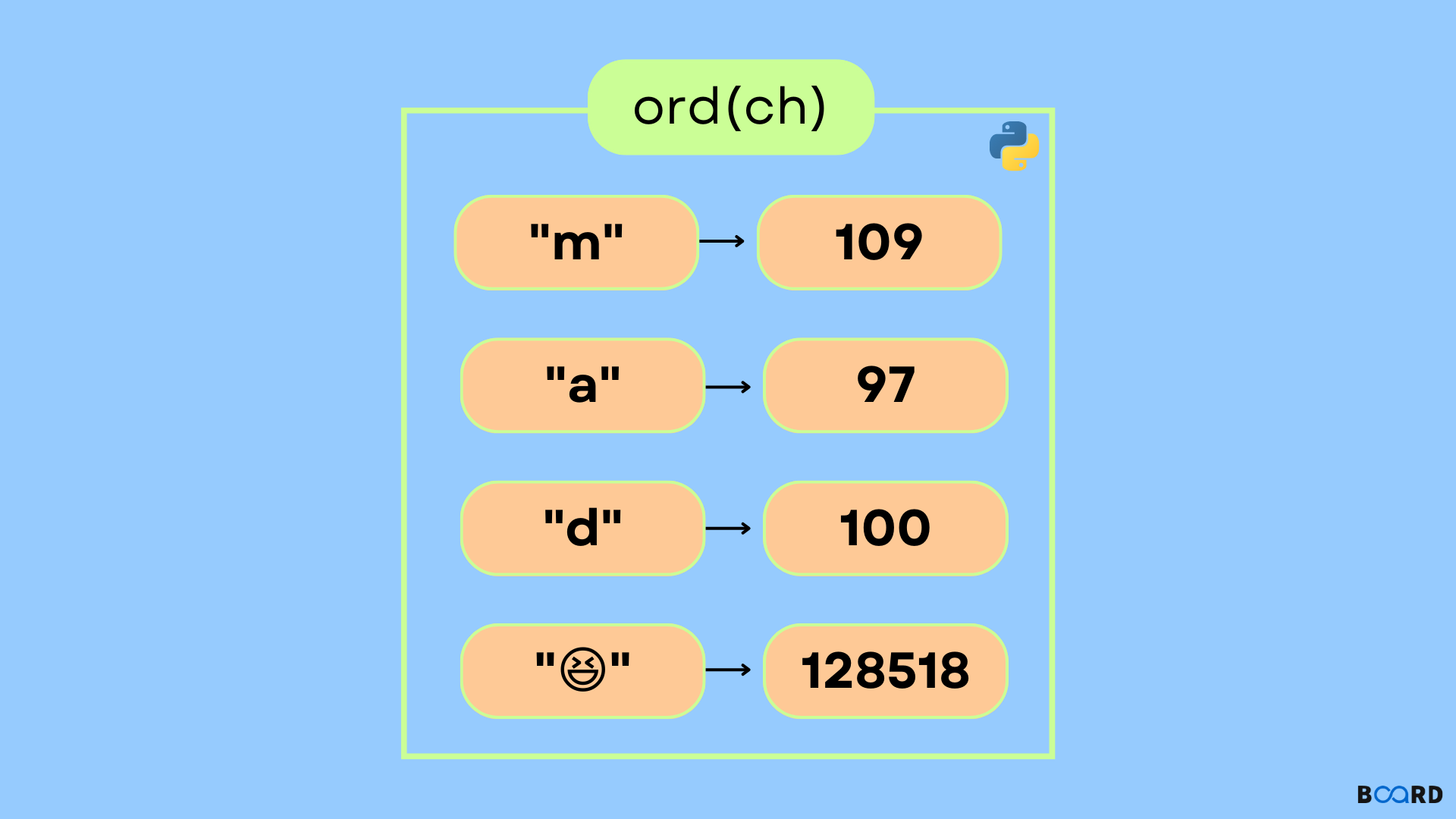# ord() in Python## Introduction

Are you using Unicode characters? You'll need the Python ord() method. By reading this article, find out what each one performs and why you should utilize it out exactly what one performs and why you should utilize it .

In this article, we'll look at various instances of utilizing Python's ord() method to convert a character to its Unicode code.  So let's get this party started!

## ord() METHOD

The ord() method returns the integer indicating the Unicode of a specific character.

SYNTAX -     ord(ch)

### Example 1

 # find unicode of Kcharacter = 'K'unicode_char = ord(character)print(unicode_char)

Output:

 75

### Example 2

Obtain the number corresponding to the character "h":

 x = ord("h")print(x)

Output:

 104

### Example 3

 print(ord('8'))    print(ord('x'))    print(ord('*'))

Output:

 5612042
write your code here: Coding Playground

## ord() Error Conditions

When the length of the string is less than one, a TypeError is thrown, as seen below:

 #Board Infinity # inbuilt function return an# integer representing the Unicode code# demonstrating exception# Raises Exceptionvalue1 = ord('BC')# prints the unicode valueprint(value1)

Output:

 Traceback (most recent call last):  File "", line 6, in TypeError: ord() expected a character, but string of length 2 found

The ord() function,  is indeed the inverse of the Python chr() method.

The chr() function returns a string containing the Unicode code point of a character.

 Syntax: chr(num)num : integer value

Where ord() techniques work in the opposite direction of the chr() function:

ord() and chr() function examples:

 # inbuilt function return an# integer representing the Unicode codevalue = ord("A")# prints the unicode valueprint (value)# print the characterprint(chr(value))

Output:

 65A
write your code here: Coding Playground